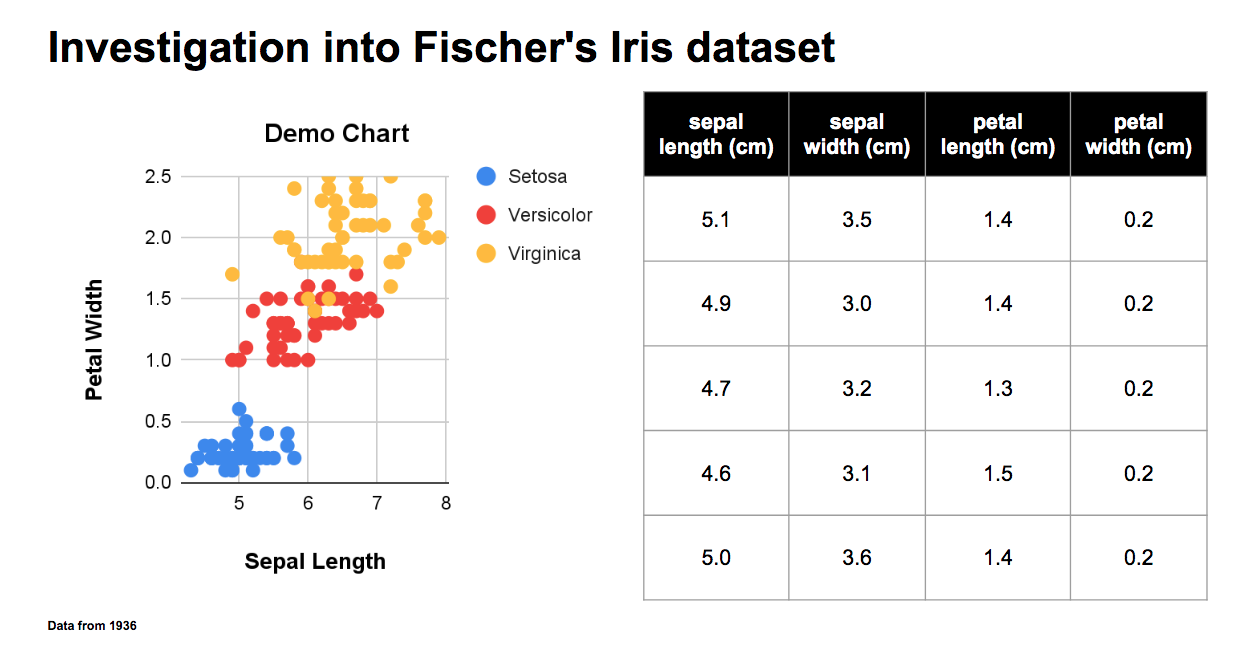# gslides: Creating charts in Google slides¶

`gslides` is a Python package that helps analysts turn `pandas` dataframes into Google slides & sheets charts by configuring and executing Google API calls.

The package provides a set of classes that enable the user full control over the creation of new visualizations through configurable parameters while eliminating the complexity of working directly with the Google API.

## Quick Installation¶

```pip install gslides
```

## Usage¶

Below is an example that only showcases a simple workflow. Full discussion around features can be found in the docs.

1. Initialize package and connection

```import gslides
from gslides import (
Frame,
Presentation,
Table,
Series, Chart
)
from sklearn import datasets
gslides.initialize_credentials(creds) #BringYourOwnCredentials
```

2. Create a presentation

```prs = Presentation.create(name = 'demo pres')
```

```spr = Spreadsheet.create(
sheet_names = ['demo sheet']
)
```

```plt_df = #Iris data
frame = Frame.create(df = plt_df,
sheet_id = spr.sheet_names['demo sheet'],
sheet_name = 'demo sheet',
overwrite_data = True
)
```

5. Create a scatterplot

```sc = Series.scatter(series_columns = target_names)
ch = Chart(
data = frame.data,
x_axis_column = 'sepal length (cm)',
series = [sc],
title = f'Demo Chart',
x_axis_label = 'Sepal Length',
y_axis_label = 'Petal Width',
legend_position = 'RIGHT_LEGEND',
)
```

6. Create a table

```tbl = Table(
)
```

7. Create a slide with the scatterplot

```prs.add_slide(
objects = [ch, tbl],
layout = (1,2),
title = "Investigation into Fischer's Iris dataset",
notes = "Data from 1936"
)
```

8. Preview the slide you have just created in your notebook

```prs.show_slide(prs.slide_ids[-1])
````gslides` also supports basic templating functionality. See this notebook for an example.

Find this Jupyter notebook detailing advanced usage of `gslides`.

## Developer Instructions¶

To install the package with development dependencies run the command

```pip install -e .[dev]
```

This will enable the following

• Unit testing using pytest - Run `pytest` in root package directory

• Pre commit hooks ensuring codes style using black and isort

• Sphinx documentation - To create sphinx run `make html` in package docs folder - To view locally run `python -m http.server`